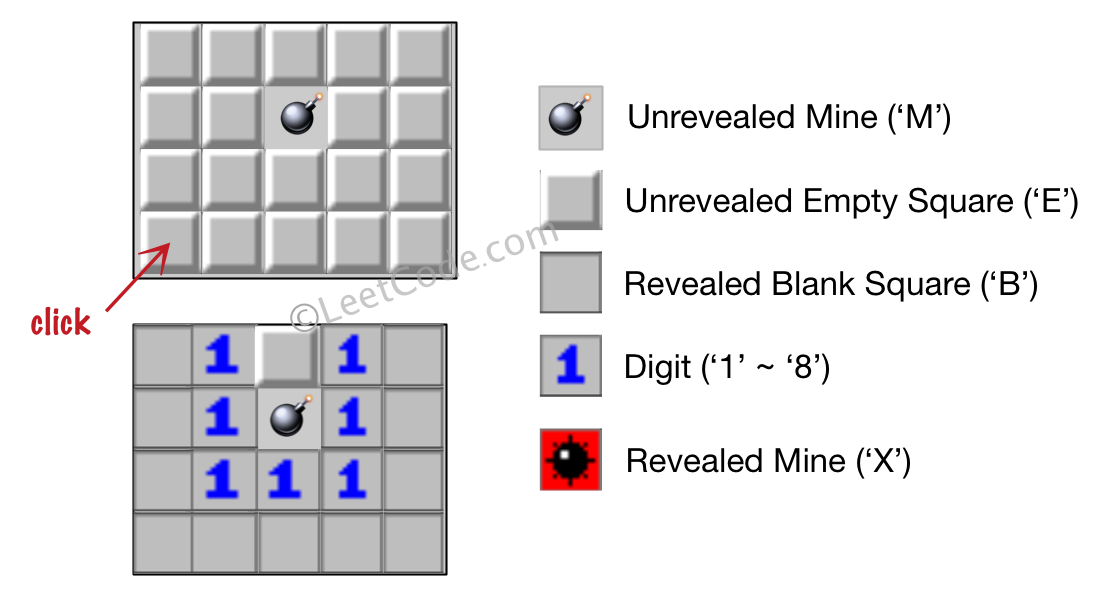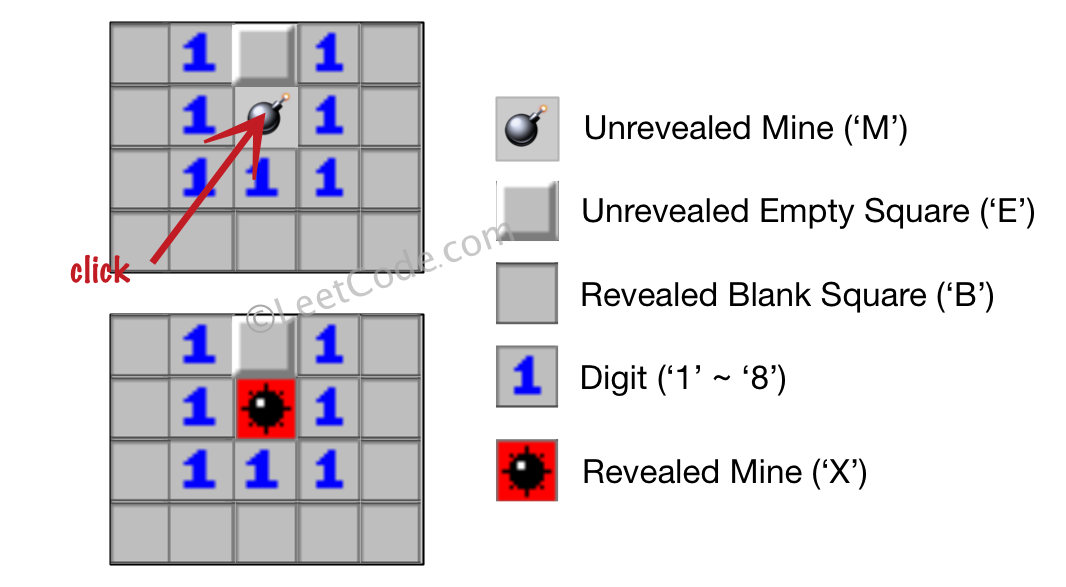529. Minesweeper
Medium
1.7K
969

Let's play the minesweeper game (Wikipedia, online game)!

You are given an `m x n` char matrix `board` representing the game board where:

• `'M'` represents an unrevealed mine,
• `'E'` represents an unrevealed empty square,
• `'B'` represents a revealed blank square that has no adjacent mines (i.e., above, below, left, right, and all 4 diagonals),
• digit (`'1'` to `'8'`) represents how many mines are adjacent to this revealed square, and
• `'X'` represents a revealed mine.

You are also given an integer array `click` where `click = [clickr, clickc]` represents the next click position among all the unrevealed squares (`'M'` or `'E'`).

Return the board after revealing this position according to the following rules:

1. If a mine `'M'` is revealed, then the game is over. You should change it to `'X'`.
2. If an empty square `'E'` with no adjacent mines is revealed, then change it to a revealed blank `'B'` and all of its adjacent unrevealed squares should be revealed recursively.
3. If an empty square `'E'` with at least one adjacent mine is revealed, then change it to a digit (`'1'` to `'8'`) representing the number of adjacent mines.
4. Return the board when no more squares will be revealed.

Example 1:```Input: board = [["E","E","E","E","E"],["E","E","M","E","E"],["E","E","E","E","E"],["E","E","E","E","E"]], click = [3,0]
Output: [["B","1","E","1","B"],["B","1","M","1","B"],["B","1","1","1","B"],["B","B","B","B","B"]]
```

Example 2:```Input: board = [["B","1","E","1","B"],["B","1","M","1","B"],["B","1","1","1","B"],["B","B","B","B","B"]], click = [1,2]
Output: [["B","1","E","1","B"],["B","1","X","1","B"],["B","1","1","1","B"],["B","B","B","B","B"]]
```

Constraints:

• `m == board.length`
• `n == board[i].length`
• `1 <= m, n <= 50`
• `board[i][j]` is either `'M'`, `'E'`, `'B'`, or a digit from `'1'` to `'8'`.
• `click.length == 2`
• `0 <= clickr < m`
• `0 <= clickc < n`
• `board[clickr][clickc]` is either `'M'` or `'E'`.
Accepted
134.9K
Submissions
205.4K
Acceptance Rate
65.7%

Seen this question in a real interview before?
1/4
Yes
No

Discussion (0)

Related Topics